What Is A Capacitor? 3
2022-12-23

Fundamentals

1. Overview

2. Capacitance

3. Dielectric Materials

4. Electric Charge

5. Electric Field

6. Impedance and Reactance

7. Parasitic Inductance

8. Q factor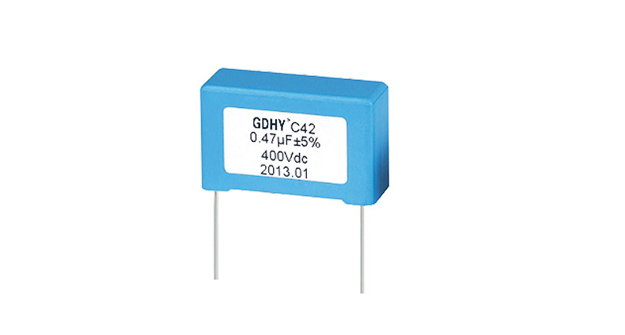6. Impedance and Reactance

Impedance and reactance

An element in a DC circuit can be described using only its resistance. The resistance of a capacitor in a DC circuit is regarded as an open connection (infinite resistance), while the resistance of an inductor in a DC circuit is regarded as a short connection (zero resistance). In other words, using capacitors or inductors in an ideal DC circuit would be a waste of components. Yet, they are still used in real circuits and the reason is that they never operate with ideally constant voltages and currents.

As opposed to constant voltage circuits, in AC circuits the impedance of an element is a measure of how much the element opposes current flow when an AC voltage is applied across it. It is basically a voltage to current ratio, expressed in the frequency domain. Impedance is a complex number, which consists of a real and an imaginary part: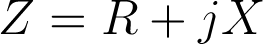where Z is the complex impedance. The real part R represents resistance, while the imaginary part X represents reactance. Resistance is always positive, while reactance can be either positive or negative. Resistance in a circuit dissipates power as heat, while reactance stores energy in the form of an electric or magnetic field.

Impedance of a resistor

Resistors in AC circuits behave the same way they do in DC circuits. Basically, the impedance of a resistor consists only of the real part, which is equal to the resistance of the resistor. Therefore, the impedance of a resistor can be expressed as: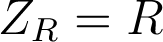where Z is the impedance, and R is the resistance of the resistor. It is obvious that a resistor has no reactance, and can therefore store no energy. Also, when a voltage is applied across the resistor, the current flowing through the resistor will be in phase with the voltage, as can be seen in this illustration: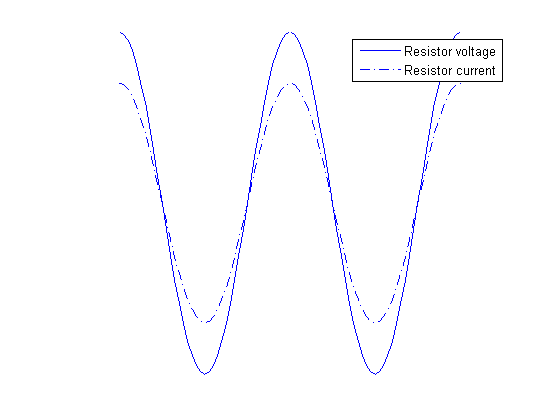Impedance of a capacitor

Capacitors are components which introduce a certain capacitance into a circuit. They are used to temporarily store electrical energy in the form of an electric field. While this definition is technically correct, it doesn’t mean much to a hobbyist or even to most engineers. It is perhaps more appropriate to say that capacitors are used to lag the voltage by 90 degrees compared to the current, in the time domain. This effect is better described graphically: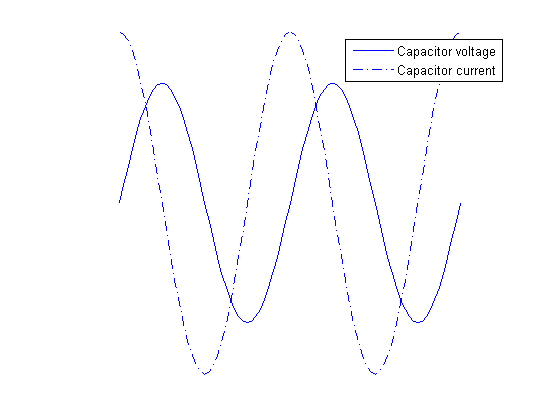As can be seen from the graph, the voltage of a capacitor lags behind the capacitor current. Alternatively, it can be said that the capacitor current leads capacitor voltage by 90 degrees. In order to represent this fact using complex numbers, the following equation is used for the capacitor impedance:where ZC is the impedance of a capacitor, ω is the angular frequency (given by ω=2πf, where f is the frequency of the signal), and C is the capacitance of the capacitor. Several facts are obvious from this formula alone:

The resistance of an ideal capacitor is infinite.

The reactance of an ideal capacitor, and therefore its impedance, is negative for all frequency and capacitance values.

The effective impedance (absolute value) of a capacitor is dependent on the frequency, and for ideal capacitors always decreases with frequency.

Impedance of an inductor

Similarly, inductors are components which introduce a certain inductance into a circuit. They are used to temporarily store electrical energy in the form of a magnetic field. Therefore, inductors are used to lag the current by 90 degrees compared to the voltage, in the time domain. The following graph explains this phenomenon: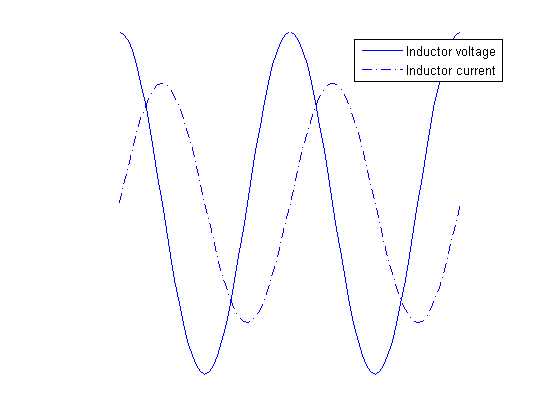The voltage of an inductor leads the capacitor current by 90 degrees. The following equation is used for the impedance of an inductor: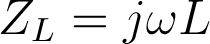where ZL is the impedance of the given inductor, ω is the angular frequency, and L is the inductance of the inductor. Again, several conclusions can be drawn from this formula:

The resistance of an ideal inductor is zero.

The reactance of an ideal inductor, and therefore its impedance, is positive for all frequency and inductance values.

The effective impedance (absolute value) of an inductor is dependent of the frequency and for ideal inductors always increases with frequency.

Ohm’s law

Ohm’s law was originally formulated for DC circuits, and it states: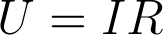To make sense for AC circuits, it was later expanded with the use of complex numbers, and the new formulation is: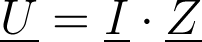where U is the complex voltage between two points, I is the complex current, and Z is the complex impedance. Since impedance is always regarded as a complex number, we have omitted the underscore for the impedance throughout this text.

The notion of complex voltages and currents can be confusing at first, so let’s try to explain this first. AC circuits are often in a steady state, where there are one or more power sources operating at the same frequency, giving a sinusoidal output. In this case, it can be proven that all the voltages and currents in the circuits are also sinusoidal waveforms oscillating, all of them oscillating at the same angular frequency, ω. However, these voltages and currents are not in phase, generally speaking. If the voltage in an AC circuit is given as a cosine wave such as this one: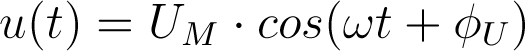where u(t) is the voltage between some two points in a circuit given as a function of time, UM is the amplitude, ω is the angular frequency, and ΦU is the phase, then the complex representation of this voltage is: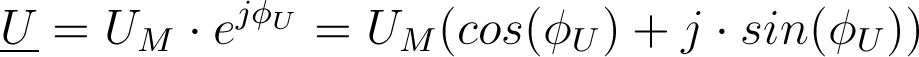On a circuit-wide scale, it is common to use one signal as the phase-reference signal. This means that it is assumed that the phase of that signal is zero, and phases of all other signals (voltages and currents) are determined in regard to that reference.

Equivalent impedances

Connection in series

If two impedances are connected in series, the equivalent impedance is obtained by simple addition - Ze=Z1+Z2. The addition of two complex is easily perfomed like this: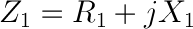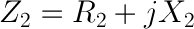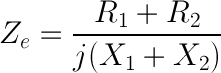For example, a 10 Ω resistor connected in series with a 1mF capacitor at 100Hz will have the equivalent impedance of: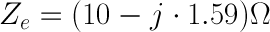The effective impedance, also called the magnitude of the impedance is calculated as: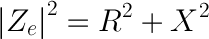In our example, the magnitude of the impedance equals:

equivalent6

Connection in parallel

To obtain the equivalent impedance of two impedances connected in parallel, we will first define admittance. The unit of admittance is a siemens [1 S] and it is the measure of how easily an element will allow current to flow, and its value is the inverse of impedance: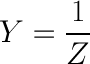The equivalent admittance of two impedances connected in parallel is equal to the sum of individual admittances: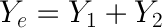If we use the same values as in the previous example, we can easily obtain: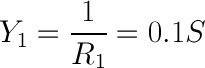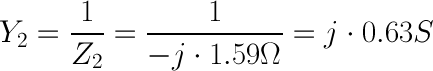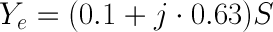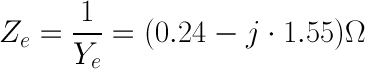This gives the impedance magnitude of: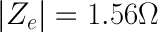7. Parasitic Inductance

What is inductance?

Electric inductance is a property of all conductors. A change in the current flowing through the conductor creates (induces) a voltage in that conductor, as well as all nearby conductors. The induced voltage opposes the change in the current that induced the voltage. Inductance is a consequence of two laws of physics. Firstly, a constant current flowing through a conductor creates a constant magnetic field. Secondly, a variable magnetic field induces a voltage in all nearby conductors, including the conductor which was used to create the magnetic field in the first place. When these two laws are combined, the resulting effect is inductance. Just like resistors are used to introduce a desired resistance in a circuit, and like capacitors are used to introduce a desired capacitance, inductors are electrical elements used to introduce a desired amount of inductance into the circuit. The inductance formula for an ideal solenoid (a coil of wire) wound around a cylindrical body of material is given as: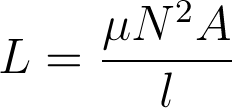where L is the inductance, µ is the magnetic permeability of the material used in the inductor, A is the cross-sectional area of the coil and l is the length of the solenoid (not the length of the wire, but the longitudinal dimension of the coil).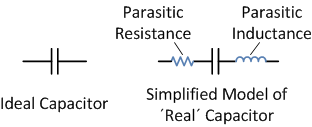An ideal capacitor has no resistance and no inductance, but has a defined and constant value of capacitance. The unit used to represent inductance is henry, named after Joseph Henry, an American scientist who discovered inductance.

Parasitic inductance

Parasitic inductance is an unwanted inductance effect that is unavoidably present in all real electronic devices. As opposed to deliberate inductance, which is introduced into the circuit by the use of an inductor, parasitic inductance is almost always an undesired effect. There are few applications in which parasitic inductance is actually a desired effect, such as helical resonators which can be used as filters. Just like all other real elements used in electronics, such as resistors or even connecting wires, capacitors exhibit this effect as well.

In order to understand the impact of parasitic inductance on a capacitor, one must first grasp the concept of reactance.

Reactance

In a DC circuit, every element can be described by its resistance. Resistors have a certain fixed amount of resistance, R. Capacitors in DC circuits can be regarded as elements with an infinite resistance (no current flowing through a capacitor), while inductors can be regarded as short connections (no voltage drop across an inductor) in a DC circuit.

AC circuits, however, are different. Each element in an AC circuit can be described by its impedance. Impedance is a measure of just how much an element “impedes, or opposes, current flow when a fixed-amplitude variable voltage is applied across its terminals.

Impedance can be further divided into two parts, called resistance and reactance. Applying a variable voltage across a purely resistive component (a resistor) in an AC circuit will result in a certain amount of alternating current flowing through the element, and this current will be in phase with the voltage. Applying a variable voltage to a purely reactive component in an AC circuit will result in an alternating current through the element, but this current will be ±90 degrees out of phase compared to the voltage, depending on which element is used - a capacitor or an inductor. Namely, the voltage across a capacitor lags behind the current by 90 degrees, while the current through an inductor lags behind the voltage across the inductor by 90 degrees. The formula for impedance is given as: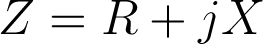where Z is the impedance, R is resistance and X is reactance. It is worth noting that impedance is a complex number, whose real part is the resistance, and imaginary part is the reactance.

Ideal capacitors and inductors are purely reactive components, and they influence only the reactive, imaginary part of impedance. However, they do so in distinct ways. The reactance of a capacitor is given as: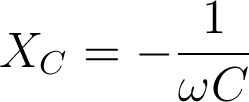where ω is the angular frequency (ω=2pf, where f is the frequency of the signal), and C is the capacitance. On the other hand, the reactance of an inductor is given as: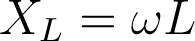where L is the inductance of the inductor. It should be noted that the reactance of a capacitor is a negative value and the reactance of an inductor is a positive value.

The effect of parasitic inductance on a capacitor

As previously indicated, the reactance of a capacitor is of opposite sign than the reactance of an inductor. This means that any parasitic inductance present on a capacitor will reduce the impedance of that capacitor by a certain amount. To illustrate this, consider the following formula: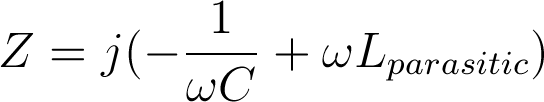where Z is the impedance of a capacitor exhibiting parasitic inductance (but not exhibiting parasitic resistance). Let’s analyze this formula in order to understand the effect of parasitic inductance on a capacitor.

Let’s assume an angular frequency of 1Mhz (approx. 6.2·106 rad/s), a capacitance of 0.1 µF and a typical parasitic inductance for ceramic capacitors, approximately 1nH. In the absence of any parasitic effects, the impedance of such a capacitor would be approximately -j·1.591 Ω. If parasitic effects are considered, the impedance is now -j·1.585 Ω. Not a big deal, since the effective impedance is only 0.37% less when a parasitic inductance is present.

However, at larger frequencies, parasitic inductance becomes a bigger problem. Let us now increase the frequency to 10MHz and repeat the calculation. The angular frequency is now approximately 6.2·107 rad/s. In the absence of parasitic effects, the impedance of a 0.1 µF capacitor would be approximately -j·0.1591 Ω. If we introduce parasitic impedance, the impedance is now -j· 0.0963 Ω. The effective impedance is now reduced by 40%! At higher frequencies, this becomes an increasing problem and at some point the impedance becomes positive and the capacitor in facts starts acting like as an inductor.

For this reason, there are low inductance capacitors specially made for high frequency applications and for applications where parasitic effects are highly undesirable. They are made using special materials and packaging with leads made as short as possible. In order to reduce inductance further, the internal layout of a capacitor is designed to cancel out all magnetic fields generated by the capacitor current.

8. Q factor

Q Factor definition

The Q factor of a capacitor, also known as the quality factor, or simply Q, represents the efficiency of a given capacitor in terms of energy losses. It is defined as: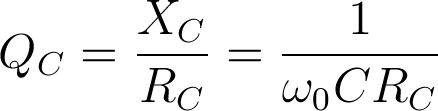where QC is the quality factor, XC is the reactance of the capacitor, C the capacitance of the capacitor, RC is the equivalent series resistance (ESR) of the capacitor, and ω0 is the frequency in radians at which the measurement is taken.

In an AC system, the Q factor represents the ratio of energy stored in the capacitor to the energy dissipated as thermal losses in the equivalent series resistance. For example, a capacitor that is capable of storing 2000 joules of energy while wasting only 1 joule has a Q factor of 2000. Since Q is the measure of efficiency, an ideal capacitor would have an infinite value of Q meaning that no energy is lost at all in the process of storing energy. This is derived from the fact that the ESR of an ideal capacitor equals zero.

The Q factor is not a constant value. It changes significantly with frequency for two reasons. The first reason is the obvious ω0 term in the above equation. The second reason is that ESR is not a constant value with regard to frequency. The ESR varies with frequency due to the skin effect, as well as other effects related to the dielectric characteristics.

A related term, called the dissipation factor(DF), is sometimes defined in capacitor datasheets instead of the Q-factor. In AC circuits the DF is simply the reciprocal value of Q.

Why is the Q factor important?

Most applications do not have to take the Q factor into serious consideration, and standard capacitors may be used in those applications. However, the Q factor is one of the most important capacitor characteristics in the design of RF circuits. At RF frequencies, the ESR increases with frequency due to the skin effect. Along with the increase in ESR, dissipative losses increase as well. This is why RF circuits typically use high-Q capacitors to reduce high-frequency losses.

Typical appications that call for high-Q capacitors are RF matching applications, MRI imaging coils used in MRI scanners and other applications that have to be precisely tuned at higher frequencies. In some applications, the losses at the capacitor itself can be high enough to actually raise the temperature enough to de-solder from the board, which is why high-Q capacitors must be used in such applications. Even if the temperature increase isn’t as drastic, it can still affect the lifetime of other neighbouring components on the board. Another reason to use high-Q capacitors is the reduced thermal noise. All real capacitors have an equivalent series resistance, and this resistance creates additional thermal noise. In applications such as satellite receivers, noise levels are critical and high-Q capacitors are used in order to maintain the desired signal-to-noise ratio.

Typical values

Datasheets usually quote the Q factor at one or more frequencies. The standard frequency used in Q factor measurements is 1MHz. However, since the Q factor varies greatly with frequency, the Q factor given at 1MHz is not a good approximation of the Q factor at, for example, 2GHz.

Some datasheets will give Q factor values at higher frequencies if the capacitor was intended for use at high frequencies. Some datasheets will even provide a Q factor vs. frequency graph which can be used to deduce the Q factor at any given frequency.

Good high-Q capacitors can have a Q factor value of over 10,000 at 1MHz and over 1,000 at 100MHz, while standard capacitors can have a Q factor as low as 50 at 1kHz. The difference between a high-Q capacitor and a standard capacitor is in the actual design of the capacitor, as as well as the materials used. All connections and pins are kept as short as possible to reduce resistance, and are made from low-resistance materials such as copper. Most high-q capacitor manufacturers offer multilayer ceramic chip capacitors, which are small and sturdy, have a long lifetime, tight tolerances and great stability over time, providing high Q values, but are often limited to a few tenths of picofarads. Such capacitors can be used at frequencies of up to 20GHz, which is sufficient for most RF applications.

At lower frequencies, for example in audio applications, tantalum capacitors may be used as high-Q capacitors. They offer low ESR at sufficiently high frequencies and can sometimes be a viable alternative if higher capacitances (up to around 1mF) are needed by the circuit design.

RECOMMENDED FOR YOU
GDHY Revolutionizing Capacitor Design to Enhance Performance and Efficiency in Small Welding and Quenching Equipment
Precautions for using film capacitors
Start capacitor in AC Induction Motor
How does metallized polyester film capacitors work in the application?
How to chose water cooled resonant capacitor ?
What is an axial film capacitor?
What is a plastic film capacitor?
IGBT Snubber Capacitors Selection Guide
What is a metallized polyester film capacitor?
What is a coupling capacitor and a bypass capacitor?
Get In Touch With Us
If you contact us now for more details, you can take samples for free. Our service team will get back to you with in 24 hours normally !